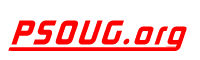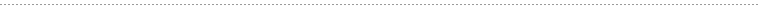Quick Search:Snippet Name: Fast random row picker two

Description: This will return a random row in about 0.05 seconds, regardless of the size of the table. If the max_id of the table is not dynamically changing, the function can be rewritten to only execute one database query instead of two.

Comment: (none)

Language: MYSQL
Highlight Mode: MYSQL

```FUNCTION random_row(\$table, \$column) {

\$max_sql = "SELECT max(" . \$column . ")

AS max_id

FROM " . \$table;

\$max_row = mysql_fetch_array(mysql_query(\$max_sql));

\$random_number = mt_rand(1, \$max_row['max_id']);

\$random_sql = "SELECT * FROM " . \$table . "

WHERE " . \$column . " >= " . \$random_number . "

ORDER BY " . \$column . " ASC

LIMIT 1";

\$random_row = mysql_fetch_row(mysql_query(\$random_sql));

IF (!is_array(\$random_row)) {

\$random_sql = "SELECT * FROM " . \$table . "

WHERE " . \$column . " < " . \$random_number . "

ORDER BY " . \$column . " DESC

LIMIT 1";

\$random_row = mysql_fetch_row(mysql_query(\$random_sql));

}

return \$random_row;
}```